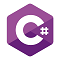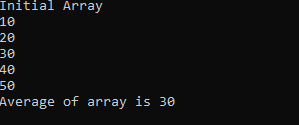# Snippets Zone#### Program to find the average of an array in C#

2994  04-July-2021

In this snippet, we will see How to find/calculate the average of an array in C#

In this snippet, we will see How to find the average of an array in C#. In order to find the average of the array, first, we need to find the sum of all array elements and then divide the sum by the length of the array.

Program to find the average of an array in C#:

``````static void Main(string[] args)
{
//Initial Array
int[] arr = { 10, 20, 30, 40, 50 };
//Variable to hold Sum and Average of Array
int sum = 0;
int average = 0;
//Print initial array values on console
Console.WriteLine("Initial Array");
foreach (var a in arr) {
Console.WriteLine(a);
//Calculate sum of array
sum += a;
}
//Calculating average
average = sum / arr.Length;
//Showing Average on the Console
Console.WriteLine("Average of array is {0}",average);
//Console.ReadLine() to hold the screen after the execution of the program